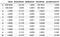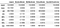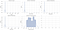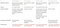# Feature Transformation in Machine Learning: Why, When and How?

Features in the dataset often need to be preprocessed before it is used in machine learning models for better model performance, which is why most of data scientists’ time is spent on data cleaning and preparation. I can think of 2 main reasons why we would want to do feature transformation:

1. Improves model performance
• How? Variability of feature due to extreme values could be reduced
Extreme values will lead to extreme estimates when modelling. A dataset with features having very different magnitude poses a problem in some machine learning algorithms as the feature with larger magnitude might be given higher weightage in comparison to features with smaller values. Distance-based algorithms like K-Nearest-Neighbours, SVM, and K-means are likely to be influenced by extreme values since the distance are calculated based on the values of the data points to determine their similarity. Feature transformation will allow these algorithm to consider each feature equally.
• How? Non-linear relationship can be modelled better
Given that the relationship between continuous variable and the target variable may not be linearly related, applying some form of transformation can model the relationship better and lead to improvement in model performance.

2. Improve numerical stability, training process and model interpretability

• Transformation can improve the numerical stability of models using gradient-descent optimisation such as linear regression, logistic regression and neural networks. It can also speed up the training process. (Read this) Hence although these models may be unaffected in model performance by scaling, there are other advantages of performing transformation.
• It is also important to note that interpretation of model coefficients can be affected by the scale of feature. In a dataset with Income and Age, Income ranges from \$0 to say, \$100K, whereas the range for Age is likely to fall between 0 to 100. Income can be a 1,000 times larger in value than Age. Given that the model parameters of a multivariate linear regression will be adjusted to counteract the scale of Income and Age, they are largely unaffected by the scale of different features,. The coefficients of Income will be compensated with a smaller coefficient as compared to Age. As such, comparison of coefficients to assess feature importance will not be meaningful.

3. Promotes robustness of model

• How? Variability of feature due to extreme values could be reduced
When we transform a feature, the skewness of the feature could be reduced and the transformed feature that goes into the model are less extreme.
• How? More resilient to changes in underlying distribution of feature
Specifically, for feature transformation by grouping numerical features (method 3 below) into several ordinal values promotes robustness as it is more resilient to changes in distribution of the feature over time.

There is information in the tails.

Side note: Should extreme values be considered as outliers and be removed? Extreme values due to measurement error should definitely be removed. Otherwise, extreme values not due to measurement error can sometimes tell us important information about the dataset. In the example of Income, assuming that the dataset contains a high net-worth individual with income of \$1M — it is definitely an extreme and rare value but also a very likely case that our model may need to be applied on for similar individuals like him/her. Excluding such data points can lose useful information for our model. My best bet is to model as is and removing them to see if it makes a difference to the model.

To preprocess numerical features, there are several methods and the most common are:

1. Transformation of feature values
2. Log-transformation of feature values
- Typically used on continuous variables that are naturally skewed with long tails of extreme values e.g. Income. By performing log-transformation, a right-skew distribution will become closer to normal.
3. Categorisation of numerical features into dummy or ordinal variables
• This method can be applied through 3 approaches: Quantiles, meaningful/arbitrary binning, automated categorisation through information gain.

This article focuses on the first method on transforming the features. Transformation of feature values usually aims to scale features into similar range/scale and can be done through Standardising and Normalising. First, what is the difference between Standardising and Normalising?

Standardising refers to transformation of a feature to obtain mean = 0 and standard deviation = 1. This is formulated by subtracting the feature values by its mean and dividing by its standard deviation. If we have a feature that follows a Gaussian distribution, by subtracting the mean and dividing by standard deviation, it gives us exactly a standard Gaussian distribution with mean 0 and standard deviation of 1.

Normalising refers to transformation of a feature to obtain a feature with values between 0 and 1, which brings the feature values to a standard scale. This is formulated by subtracting the feature value by its minimum value and dividing it by the range of the feature.

# Why and when do you standardise?

Standardising is important when we compare different attributes that have very different units. Attributes that do not have similar scale will contribute differently to the model and may end up creating a bias. Transforming the data into comparable scales will prevent this issue from happening.

This technique assumes the data to have a Gaussian distribution. While this assumption does not need to be strictly true, standardisation is useful when applied in models that assumes the data to follow Gaussian distribution. Examples of such models are linear regression and logistic regression.

Standardisation does not have a bounded range i.e. min/max like normalisation do. This meant that outliers are not as affected by outliers as compared to normalisation and the model can be more robust. However, it should be noted that though standardisation performs slightly better than normalisation in the presence of outliers, outliers will still skew the feature to some extent after standardisation since the mean and standard deviation takes into account these outlier values.

# Why and when do you normalise?

Normalising is important when we have attributes of very different range. Normalisation helps to transform the attributes into a common scale with fixed maximum and minimum values.

In contrast to standardisation, normalisation does not require the assumption of Gaussian distribution. Normalisation is useful when the data has varying scales and the model to be applied does not assume the underlying distribution of the data.

Normalising your data will scale the data to an interval between 0 to 1. The presence of outliers will skew the transformation towards the extreme value, leaving the inliers to be very similar and small.

# Dealing with Extreme Values

Given that both Standardisation and Normalisation can be skewed by outliers through the mean, standard deviation, min and max values, there is a robust standardisation technique we could apply. Robust standardisation scales the values using its median and interquartile range and are therefore not influenced by few large/small values. This way, extreme values are not taken into account in the transformation.

Do note that even robust scaling are not immune to outliers and they will not be “removed”. If the goal is to have an outlier clipping i.e. binned at the extreme values, a non-linear transformation is required, perhaps using `QuantileTransformer` as suggested in scikit-learn docs.

Here’s an overview of what `QuantileTransformer` does:

1. An estimation of the CDF distribution of the feature is computed
2. and used to map the original values into a uniform distribution (in other words, frequent values are spread out into uniform distribution) or normal distribution (parameter specified in function)
3. The obtained values is mapped to the desired output distribution using the associated quantile function

Feature values that fall above or below the range of the seen data will be mapped to the bounds of the fitted distribution. Since this scaler changes the underlying distribution of the variables, linear relationships among variables may be destroyed by using this scaler. Thus, it is best to use this for non-linear data.

# Illustration

For illustration, I’m using the sample Iris dataset from seaborn to get some random values and selected `sepal_length` to be used as the `original` raw data to be transformed. To simulate the presence of outliers, I've replaced the first two values with extreme values of 10,000 and 5,000, about 1000x the mean of the column. After applying the various techniques as discussed, the results can be seen below.Preview of original data vs other transformation techniques

Observation 1: Both transformed columns `StandardScaler` (standardisation) and `MinMaxScaler` (normalisation) are heavily skewed by the extreme values, which leaves the inliers data points to be very similar to one another. Particularly for MinMaxScaler case, we can only observe the variation in values at 5 d.p.

Observation 2: With `RobustScaler`, the inliers are more varied and are less influenced by the extreme values. We should also note that the extreme values after transformation are still large in magnitude (rmbr that robust scaling is not immune to outliers!).

Observation 3: Lastly, we have a winner! `QuantileTransformer` performs the best with outliers. Overall, the transformed values lie between 0 and 1. Outliers take on values closer to 1, while inliers are more differentiable as compared to the other `MinMaxScaler`.Descriptive stats on various transformation techniques

Distribution plots quickly confirm our observations. In the first row, we observe that majority of the data points are clustered close to 0 with some extreme values on the positive end. The x-axis as narrower as we apply transformation from standardisation to normalisation. Quantile transformer on the other hand, shows us a more uniform distribution with values between 0 and 1.Distribution plots of various transformation techniques

Here’s a side by side comparison of all the techniques discussed.Summary

# Other methods of transformation

(This article talks about other transformation techniques!)

1. `from sklearn.preprocessing import MaxAbsScalar` - scales by dividing the values by the maximum absolute value of the feature.
2. `from sklearn.preprocessing import PowerTransformer` - also changes the distribution of the feature to be more Gaussian
• Automates the decision of square root/cube root/log transformation by introducing a parameter lambda and finds the best value of lambda based on Box-Cox transformation or Yeo-Johnson transformation.

# Final Notes

1. General questions we could ask ourselves before deciding which transformation technique to apply (if needed at all):
• What algorithms are we applying? Does the algorithm we are applying assume any distribution on data?
- Tree-based algorithms are insensitive to the scale of the features given that they are a rule-based algorithm. A node of a decision tree splits by maximising the information gain of a single feature and is not influenced by other features. Scaling this specific feature or scaling the others will not affect the information gain and homogeneity of the node. Hence they are invariant to the scale of features and are robust to outliers.
- Distance-based algorithms like K-Nearest-Neighbours, SVM, and K-means are likely to be influenced by extreme values. Feature transformation will allow these algorithm to consider each feature equally.
- Machine learning algorithms such as linear regression, logistic regression, neural network, etc. that use gradient descent as an optimisation technique can improve the numerical stability and training process.
- Algorithms such as K-nearest neighbours and neural networks does not assume any distribution, which is great for normalisation to be applied.
• Does the feature follow a Gaussian distribution?
- Normalisation is great when the feature doesn’t follow Gaussian distribution.
• Are there outliers/extreme values in the feature?
- Robust scaling and quantile transformer are great techniques in this case.

2. While there can be general guides to help us decide which transformation technique to apply, most of time it can be helpful to try all of them and see which gives a better model performance and/or gives better model interpretation.

3. To avoid data leakage during model testing, it is best to fit and transform on training data then use it to transform on testing data.

This piece turned out longer than I intended it to be! Hope it was helpful to some of you! 🙂

I write to understand.

## More from Isabella

I write to understand.